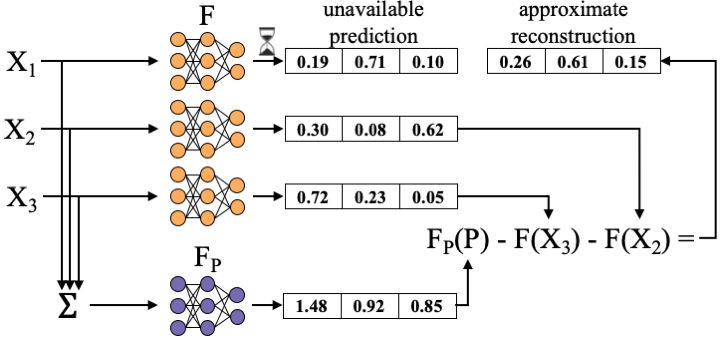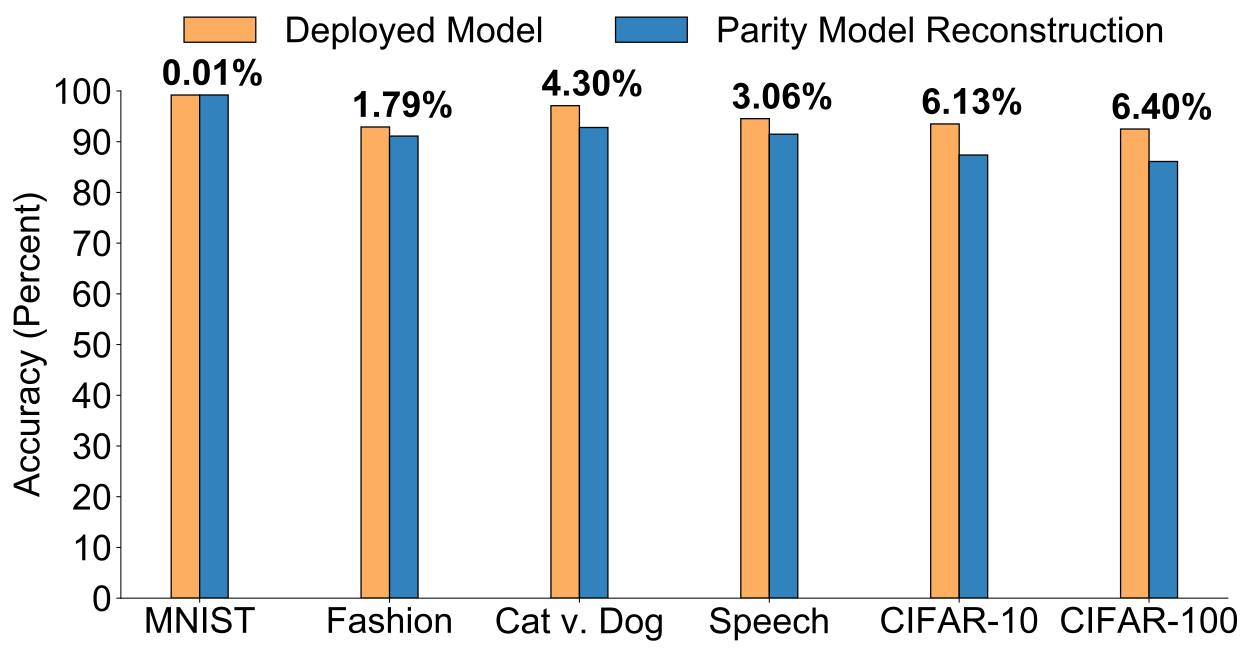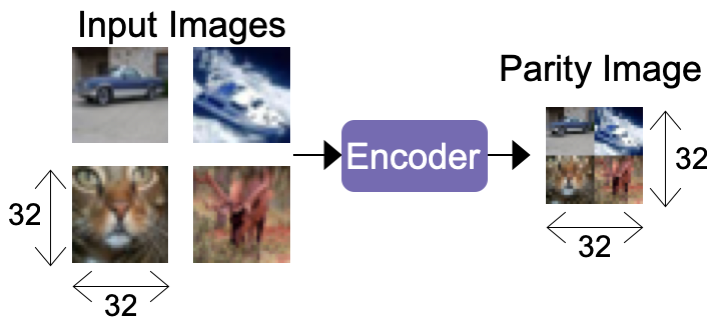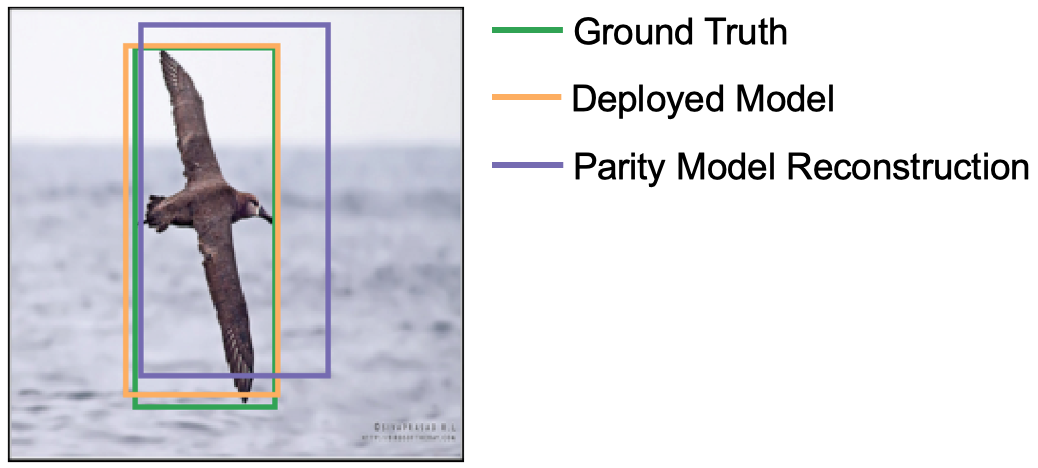In the previous post, we provided a background on coded computation and its challenges, described the potential for machine learning to advance the state-of-the-art in coded computation, and began discussing our recent work toward leveraging machine learning to extend the reach of coded computation to general nonlinear functions. In the previous post, we discussed one of the two paradigms of using learning to design coded-computation schemes: learning encoders and decoders. In this second post, we’ll describe an alternate approach to learning-based coded computation: using simple encoders and decoders, and learning computations that are performed over parities. We’ll conclude by discussing avenues for future research on learning-based coded computation. We’ll also show how simple it is to start exploring these future directions using the codebase that we have made available on Github.

## Paradigm 2: Learning a parity computation

While the approach described in Part 1 of learning encoders and decoders shows the promise of taking a learning-based approach to coded computation, it comes with one potential practical downside: neural networks can be computationally expensive. If the neural network encoders and decoders used in the approach in Part 1 add significant latency to the path of reconstructing unavailable function outputs, they may not be effective in alleviating slowdowns.

To overcome this potential practical concern, we propose a fundamentally new approach to coded computation. Rather than designing new encoders and decoders, we propose to use simple, fast encoders and decoders (e.g., summation/subtraction, concatenation). For example, a simple summation encoder used for image classification tasks would construct a parity by performing pixel-wise summation across the k input images. Similarly, a simple subtraction-based decoder would attempt to reconstruct unavailable function outputs by subtracting available function outputs from the output of the computation operating over the parity.

To enable a simple encoder and decoder pair to impart resilience over nonlinear functions, we design a new computation that operates over parities, rather than operating over parities using another copy of the original function. Within the context of machine learning inference, this new computation is a separate model, which we call a “parity model,” and denote as FP. The challenge of this approach is to design a parity model that enables accurate reconstruction of unavailable function outputs. We address this by designing parity models as neural networks, and learning a parity model that enables a simple encoder and decoder pair to reconstruct slow or failed function outputs.

By learning parity models and using simple, fast encoders and decoders, this approach is able to impart resilience to nonlinear computations, such as neural networks, while operating with low latency.

Given a fixed encoder E and decoder D, for a given function F, a parity model FP is trained through standard iterative optimization algorithms, such as stochastic gradient descent. The data input and desired output pairs used in training a parity model are generated using the properties of the encoder E and decoder D. Each input for training the parity model is a parity that results from encoding k samples from an underlying dataset (e.g., CIFAR-10) using the encoder E. For example, using a summation encoder with k = 3 and r = 1, a single input for training a parity model would be P = X1 + X2 + X3 for randomly selected X1, X2, and X3, and with summation being performed pixel-wise.

The desired output to be used in training a parity model on this input is simply the output that would enable the decoder D to accurately reconstruct one of the k outputs among F(X1), F(X2), …, F(Xk). For example, using a simple subtraction decoder parameterized with k = 3 and r = 1, the decoder would attempt to reconstruct the unavailable F(X1) as FP(P) - F(X2) - F(X3). For this decoding to exactly reconstruct one unavailable function output, it is required that FP(P) = F(X1) + F(X2) + F(X3). Thus, for sample P = X1 + X2 + X3, the desired output is the summation of the outputs of model F: FP(P) = F(X1) + F(X2) + F(X3).

Given this structure of inputs to the parity model and desired outputs from the parity model, the parity model is trained using the same iterative optimization procedure commonly used in training neural networks: perform a forward pass over FP using generated input P, compare the output of the parity model to the desired output using a selected loss function (e.g., mean squared error), and backpropagate the loss value to update the parity model.

The figure below shows an example of the parity models framework being used at inference time to reconstruct the prediction resulting from a single slow or failed server.The framework surrounding parity models opens up a rich space for exploring the design of encoders, decoders, and parity models. To showcase the ability of the framework to use simple encoding and decoding functions, we have primarily evaluated the use of parity models using summation/subtraction as the encoding/decoding functions, as described above. We have also kept the neural network architecture of the parity model the same as the original model over which it imparts resilience. However, we’ve also experimented with encoder designs that are more specific to the learning task at hand, such as an encoder specialized for image classification that downsamples and concatenates k images into a single parity. Full details on our exploration of this design space may be found in the paper: Parity Models: Erasure-Coded Resilience for Prediction Serving Systems.

We have evaluated the accuracy of using parity models along with simple encoders and decoders on a variety of tasks such as image classification, speech detection, and object localization. As shown in the figure below, across a variety of datasets, we find that using this approach with k = 2 and r = 1 leads to reconstructed predictions with an accuracy at most 6.4% lower than if the original model’s predictions (“Deployed Model” in the figure) were always available.These results show the promise of the learning-based approach to overcoming the challenge of nonlinear functions in coded computation.

With higher values of parameter k, more inputs are encoded together into a single parity, and we generally find that the accuracy of reconstructions decreases; for example the accuracy of reconstructions on the Google Speech Commands dataset with values of k of 2, 3, and 4 are 91.5%, 83.9%, and 75.6%, respectively.

In addition to the simple summation encoder we described above, we have also experimented with using encoders that are tailored to the particular inference task at hand. As an example of this, we have experimented with an encoder that is specific to image classification tasks that downsamples and concatenates k images into a single parity image as input to a parity model. An example of this encoder is shown below for parameters k = 4 and r = 1 on the CIFAR-10 dataset.Using this task-specific encoder improved the accuracy of reconstructions when using parity models on the CIFAR-10 dataset by 2% and 22% at values of k of 2 and 4, respectively. This large increase in accuracy by using a task-specific encoder shows the promise of designing encoders and decoders that are tailored to the specific inference task at hand. Later on, we’ll discuss a bit more about future directions in this space.

Finally, we have also evaluated the use of parity models in object localization, a regression task in which the goal is to predict the bounding box surrounding an object of interest in an image. The figure below shows an average example of the bounding box returned through a parity model’s reconstruction compared to that which would be returned by the original model if it were available (“Deployed Model” in the figure), as well as the ground-truth bounding box. The parity model’s reconstruction captures the gist of the localization task, and would serve as a reasonable approximation in the face of unavailability.Beyond the learning aspects of learning-based coded computation, we have also evaluated the systems-side benefit of using parity models to reduce tail latency in neural network inference. We have integrated the parity models framework into Clipper, an open-source prediction serving system, and illustrated the ability of parity models to reduce tail latency by up to 48% in the presence of resource contention. More details on the design and implementation of this system may be found in our paper, and the source code for the system evaluation in our Github repo.

### Codebase for experimenting with parity models

Implementing different types of encoders and decoders is straightforward in our framework made available on Github. Similar to the learned encoders and decoders described in the previous post, the simple encoders and decoders are also implemented as PyTorch nn.Modules. However, as these encoders and decoders are not intended to be learned, they do not contain any neural network modules within them. As illustrated in the following code snippet, the summation encoder simply uses the forward pass of the PyTorch module to perform summation:

import torch
from torch import nn

from coders.coder import Encoder, Decoder

"""
"""
def __init__(self, ec_k, ec_r, in_dim):
super().__init__(ec_k, ec_r, in_dim)

def forward(self, in_data):


You can directly run an example of using the summation encoder and subtraction decoder by following a few simple instructions. This should take less than 5 minutes on a laptop!

You can also run an example of using the concatenation-based encoder described above by following the same instructions, but using the mnist_concat.json configuration file.

## Further exploration of learning-based coded computation

Our experience with leveraging machine learning to design coded computation schemes for nonlinear functions has been positive. This work has opened up several avenues for future exploration that we believe have the potential to further push the envelope of coded computation. We now describe these promising future directions as well as show how one can easily begin exploring them using the codebase for our framework available on Github.

### Designing application-specific encoders and decoders

There are many potential encoder and decoder designs that could be employed within the parity models framework. Our evaluation results show that using a simple, general summation encoder and subtraction decoder can accurately reconstruct predictions from a variety oflearning tasks such as image classification, speech recognition, and object localization, for smaller values of k. However, there is significant room for improvement in accuracy for higher values of k. Our evaluation results have also shown that significant accuracy improvements can be achieved by designing encoders and decoders that are specific to the application at hand, such as the concatenation-based encoder described above. We believe that further exploring the design space for task-specific encoders and decoders is a promising avenue for further improving accuracy in learning-based coded computation systems.

The framework we’ve developed makes it easy to experiment with such encoders. For example, adding the concatenation-based encoder to the parity models framework required writing only around 30 lines of source code. More instructions on how to add a new encoder or decoder to the framework can be found here.

### Joint learning of encoders, decoders, and parity models

Thus far, we have considered applying machine learning to disjoint sets of the operations that occur in coded computation: the encoder, the decoder, and the computation over parities. First, in Part 1, we explored learning encoders and decoders, while keeping the computation over parities the same as that used by the original computation. Next, we explored leveraging a simple encoder and decoder pair, and instead learning a computation that takes place over parities. In general, learning-based coded computation can be extended to jointly learn all of these components. We hope that such joint optimization may help improve accuracy of reconstructions while potentially reducing the computational overhead of learned encoders and decoders.

Experimenting with such joint learning is immediately possible in the codebase of the parity models framework. Each training configuration specifies whether an encoder, decoder, and parity model should be trained using boolean flags train_encoder, train_decoder, and train_parity_model. Changing one of these flags changes whether that particular component will be learned. For example, our experiments in learning encoders and decoders, such as with the mnist_learned.json configuration file set these parameters as follows:

"train_encoder": true,
"train_parity_model": false,
"train_decoder": true


One can easily begin experimenting with joint learning of encoders, decoders, and parity models in this setup by simply changing train_parity_model to true. This also enables easy experimentation with other combinations of learned components, such as learning only a decoder. We hope that this simple configuration will enable the community to easily explore this rich design space.

## Conclusion

As distributed computing further pervades into both production and scientific computing, there is a growing need to provide resource-efficient tolerance of transient system slowdowns and failures. Coded computation offers the promise of the resource-efficient resilience that coding theory has brought to communication and storage systems. However, the inability to handle general nonlinear functions efficiently limits the reach of existing approaches to coded computation. In this blog post we have shown the potential of learning-based approaches to extend the reach of coded computation to broader classes of computations.

We are excited by the future prospects of learning-based coded computation and the synergy it brings between coding theory, machine learning, and systems research. The framework that we have developed and shared for exploring learning-based coded computation strives to make it easy for future exploration to take place. We hope that this resource enables the community to make further progress on coded computation at a rapid pace.

## References to papers described in Part 2

Learning-Based Coded Computation. Jack Kosaian, K. V. Rashmi, and Shivaram Venkataraman. In IEEE Journal on Selected Areas in Information Theory, 2020.

Parity Models: Erasure-Coded Resilience for Prediction Serving Systems. Jack Kosaian, K. V. Rashmi, and Shivaram Venkataraman. In Proceedings of the 27th ACM Symposium on Operating Systems Principles (SOSP), 2019.

• This paper contains a more detailed description of learning parity models and the systems aspects of the parity models framework (including a more detailed evaluation of latency reduction), than the journal version above.